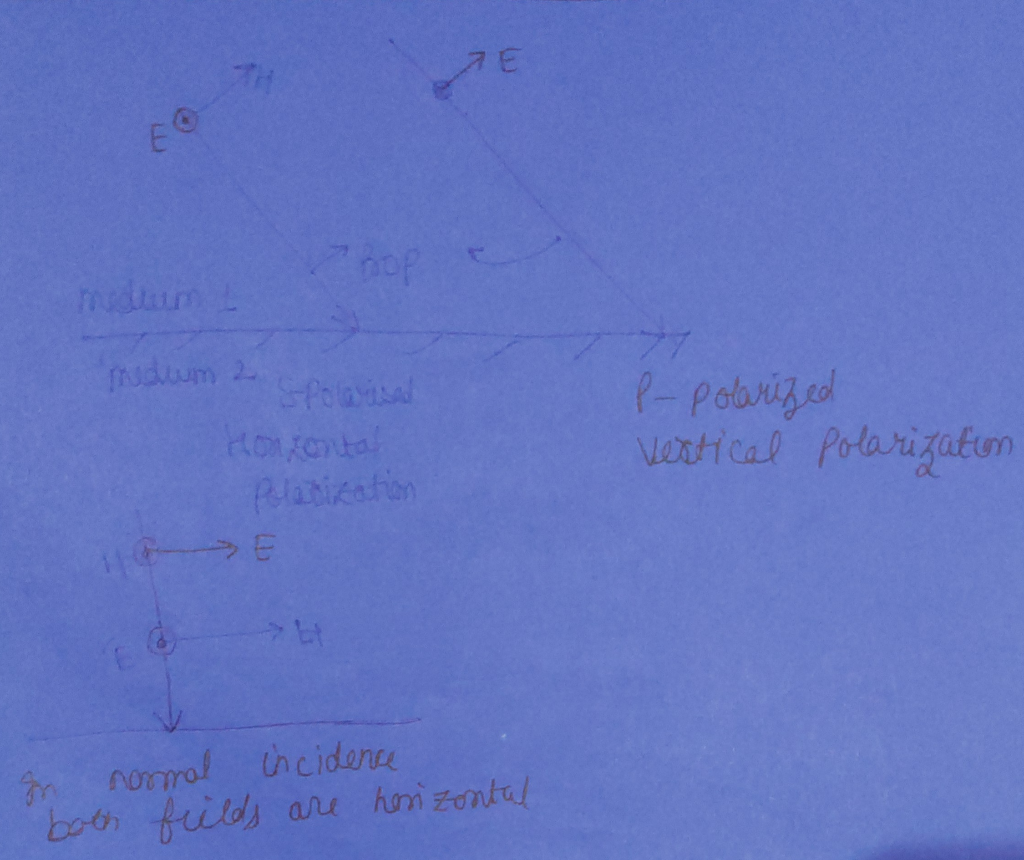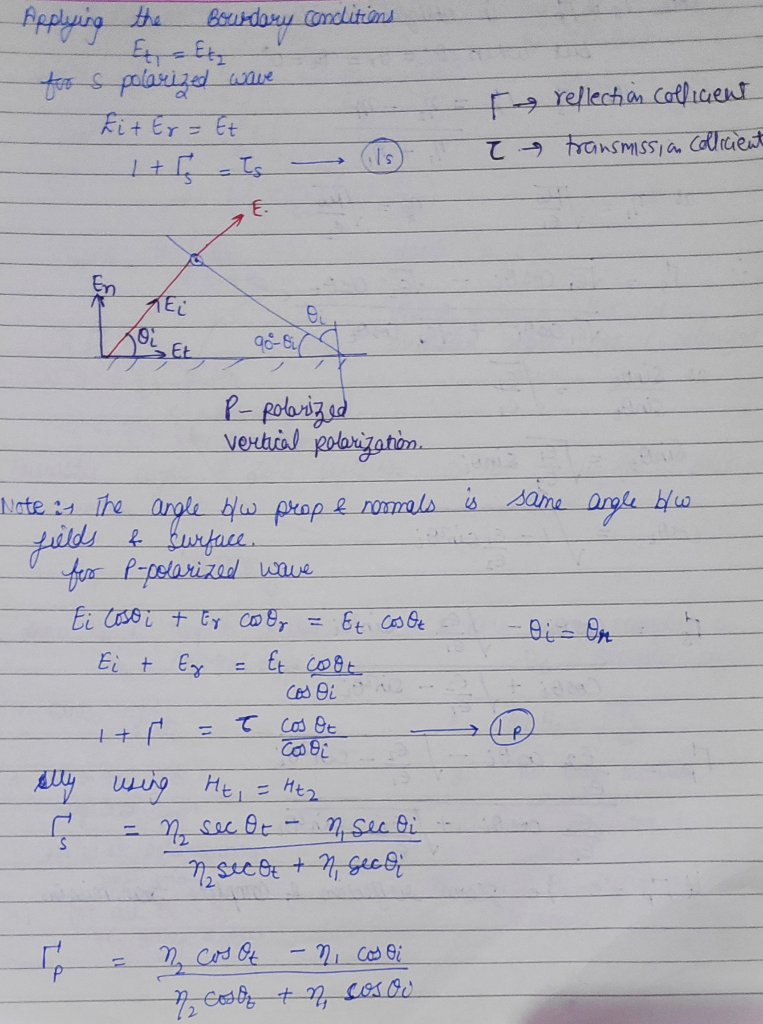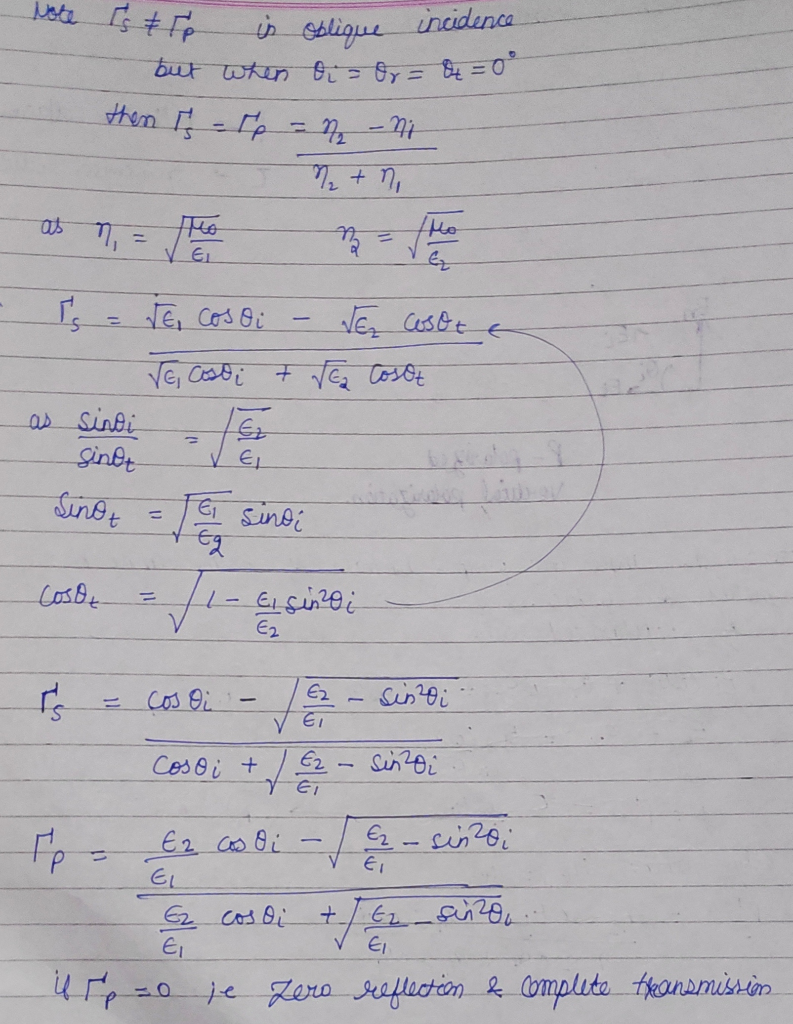##### Question

In: Electrical Engineering

# Derive the reflection coefficient and transmission coefficient for the parallel polarization. Hint: Refer the perpendicular polarization.

Derive the reflection coefficient and transmission coefficient for the parallel polarization. Hint: Refer the perpendicular polarization.

## Solutions

##### Expert Solution123

## Related Solutions

##### Determine the reflection coefficient R and the transmission coefficient T for a vacuum/plasma interface (take the...
Determine the reflection coefficient R and the transmission coefficient T for a vacuum/plasma interface (take the incident wave on the vacuum side).
##### Derive the angular spectrum of the field transmitted through an amplitude grating that the transmission coefficient...
Derive the angular spectrum of the field transmitted through an amplitude grating that the transmission coefficient is T=T0* cos^2(2pi*x/a). Assuming normal incident plane wave at wavelength ?. Based on the result, design a amplitude grating that has uniform power distribution into three diffraction orders.
##### <Quantum tunneling> State and derive transmission probability
<Quantum tunneling> State and derive transmission probability
##### In the Figure, a beam of light, with intensity 43 W/m2 and polarization parallel to a...
In the Figure, a beam of light, with intensity 43 W/m2 and polarization parallel to a y axis, is sent into a system of two polarizing sheets with polarizing directions at angles of θ1 = 70o and θ2 = 90o to the y axis. What is the intensity of the light transmitted by the two-sheet system?
##### whatis the secific meaning for eachone transmission ,reflection and refraction a diffraction .
whatis the secific meaning for eachone transmission ,reflection and refraction a diffraction .
##### Two polarizers are set up so that their polarization directions are parallel and vertical. Which of...
Two polarizers are set up so that their polarization directions are parallel and vertical. Which of the following describes light that has passed through both filters? A. The light is polarized in the veritcal direction B. the light is polarized in the horizontal direction C. The light is not polarized D. No light passes through the filters
##### Using Larmor's formula, explain why there is no reflected light for the "p" polarization (parallel to...
Using Larmor's formula, explain why there is no reflected light for the "p" polarization (parallel to the incidence plane) after a total polarization by reflection in the Brewster angle.
##### Write equations of the lines through the given point parallel to and perpendicular to the given...
Write equations of the lines through the given point parallel to and perpendicular to the given line. 4x + 6y = 0, (7/8,3/4) (a) parallel to the given line (b) perpendicular to the given line
##### How can you determine if two lines are parallel, perpendicular, or neither ?
How can you determine if two lines are parallel, perpendicular, or neither ?
##### Polarization Question (a) Vertically polarized light is incident on a polaroid sheet with its transmission axis...
Polarization Question (a) Vertically polarized light is incident on a polaroid sheet with its transmission axis at 45 degrees to the vertical. What fraction of the incident intensity emerges through the polaroid sheet? How is this emerging light polarized? (b) The light emerging from the polaroid sheet in (a) is now incident on a second polaroid sheet, with its transmission axis horizontal. What fraction of the intensity incident on the second sheet emerges? What fraction is this of the original...